# 统计机器学习 15：贝叶斯分类

## 墨尔本大学 COMP90051 课程笔记

Posted by YEY on November 20, 2019

# Lecture 15 贝叶斯分类

## 主要内容

• 离散设定下的贝叶斯思想
• Beta-Binomial 共轭
• 贝叶斯分类
• 非共轭的渐进必要性

## 1. 离散设定下的贝叶斯思想

### 1.1 如何将贝叶斯观点应用于离散数据？

• 优先考虑 生成 输入的 生成式模型
• 对比 判别式模型，其将输入作为 条件

生成式模型 判别式模型
对输入 $\boldsymbol x$ 和标签 $y$ 的联合分布 $p(\boldsymbol x,y)$进行建模，并通过贝叶斯规则来计算 $p(y\mid \boldsymbol x)$，然后选择最可能的标签 $y$ 进行预测 直接对后验条件概率 $p(y\mid \boldsymbol x)$ 建模，或者从输入 $\boldsymbol x$ 学习一个直接映射到类别的标签 $y$
例如：朴素贝叶斯 例如：Logistic 回归
• 为了便于理解，我们从最基础的设定开始
• 抛 $n$ 次硬币，其中有 $k$ 次正面朝上
• 只有 $\boldsymbol x$（结果的序列），没有 “类别” $y$
• 在离散数据上应用 生成式模型

### 1.2 离散共轭先验：Beta-Binomial

• 离散空间也存在共轭先验
• 考虑抛 $n$ 次硬币，其中有 $k$ 次正面朝上
• 令单次抛掷正面朝上的概率为 $p(Head)=q$（伯努利分布
• 推断问题为：硬币是否是公正的（即 $q \approx 0.5$）？
• 多次尝试，利用 二项式分布

$p(k|n,q)= {n\choose k}q^k(1-q)^{n-k}$
• 以及它的共轭先验 Beta 分布

\begin{align} p(q) &= \text{Beta}(q;\alpha,\beta) \\ &= \dfrac{\gamma(\alpha+\beta)}{\gamma(\alpha)\gamma(\beta)}q^{\alpha-1}(1-q)^{\beta-1} \end{align}

### 1.3 Beta 分布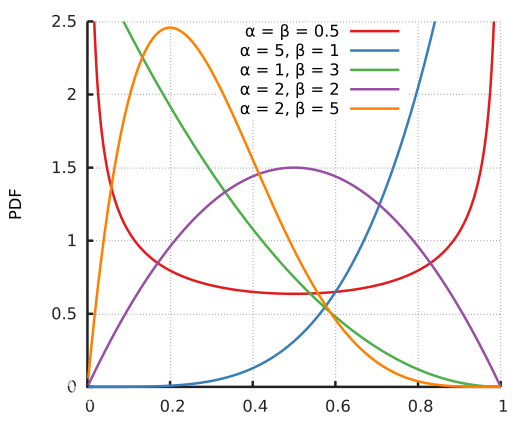### 1.4 Beta-Binomial 共轭

• 二项式（Binomial）分布

$p(k|n,q)= {n\choose k}q^k(1-q)^{n-k}$
• Beta 分布

\begin{align} p(q) &= \text{Beta}(q;\alpha,\beta) \\ &= \color{red}{\dfrac{\gamma(\alpha+\beta)}{\gamma(\alpha)\gamma(\beta)}}q^{\alpha-1}(1-q)^{\beta-1} \qquad \color{red}{\text{很好，现在我们知道了 Beta 分布的归一化算子}} \end{align}
• 证明二者共轭：

$\begin{eqnarray} \color{green}{\text{贝叶斯后验} \qquad p(q|k,n)}\; &{\color{purple}\propto}& p(k|n,q)p(q) \\ &{\color{purple}\propto}& q^k(1-q)^{n-k}q^{\alpha-1}(1-q)^{\beta-1} \\ &{\color{black}=}& q^{k+\alpha-1}(1-q)^{n-k+\beta-1} \\ &{\color{purple}\propto}& \color{red}{\text{Beta}}(q;k+\alpha,n-k+\beta) \qquad \color{purple}{\text{技巧：忽略常数项因子（归一化算子）}} \end{eqnarray}$

### 1.5 归一化的唯一性

• 我们多次用到了一种技巧： 当一个非规范分布（unnormalized distribution）和某一已知分布成比例时，我们可以认为其就是该分布。
• 如果 $f(\theta) \propto g(\theta)$，其中 $g$ 为某一已知分布，那么 $\dfrac{f(\theta)}{\int_{\boldsymbol \Theta} f(\theta)\,\text{d}\theta}=g(\theta)$
• 证明： $f(\theta) \propto g(\theta)$ 意味着

$f(\theta) = C\cdot g(\theta) \tag{1}$ $\int_{\boldsymbol \Theta} f(\theta)\,\text{d}\theta = C\cdot \int_{\boldsymbol \Theta} g(\theta)\,\text{d}\theta = C \tag{2}$

将 $(1)$ 和 $(2)$ 的等号两边的对应项相除，即可得到

$\dfrac{f(\theta)}{\int_{\boldsymbol \Theta} f(\theta)\,\text{d}\theta}=g(\theta)$

### 1.6 拉普拉斯的日出问题• 利用 Beta-Binomial 共轭，其中 $q$ 为太阳早上升起的概率 $\Pr($ “太阳早上升起” $)$
• 后验 $p(q|k.n)=\text{Beta}(q;k+\alpha,n-k+\beta)$
• $n=k=$ 观察者的年龄（按天数计算）
• 令 $\alpha=\beta=1$（等同于均匀分布先验）
• 基于上面这些假设，可以得到后验

$p(q|k)=\text{Beta}(q;\color{purple}{k+1},\color{purple}{1}) \qquad \color{purple}{\text{“自然地”计算太阳升起 / 没有升起的天数}}$

所以，明天早上太阳升起的概率的期望为

$E_{p(q|k)}[q]=\dfrac{k+1}{k+2}\qquad\qquad$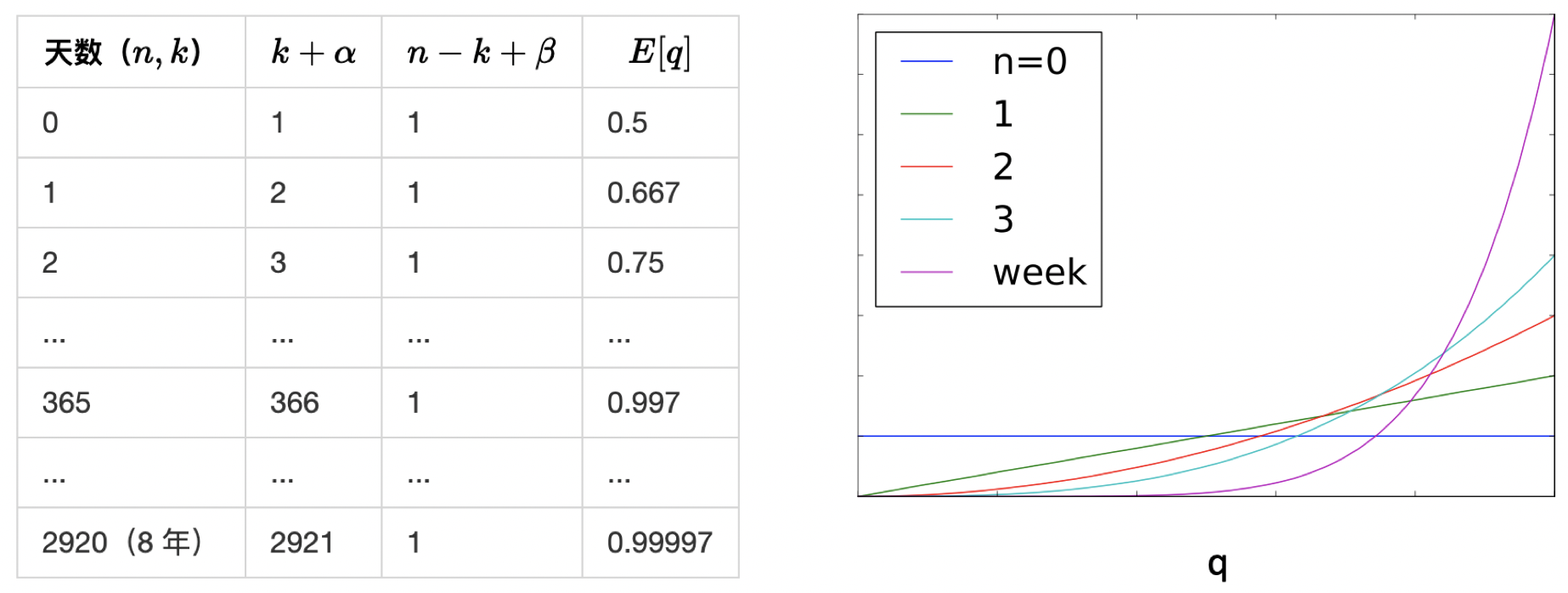### 1.7 常见的共轭先验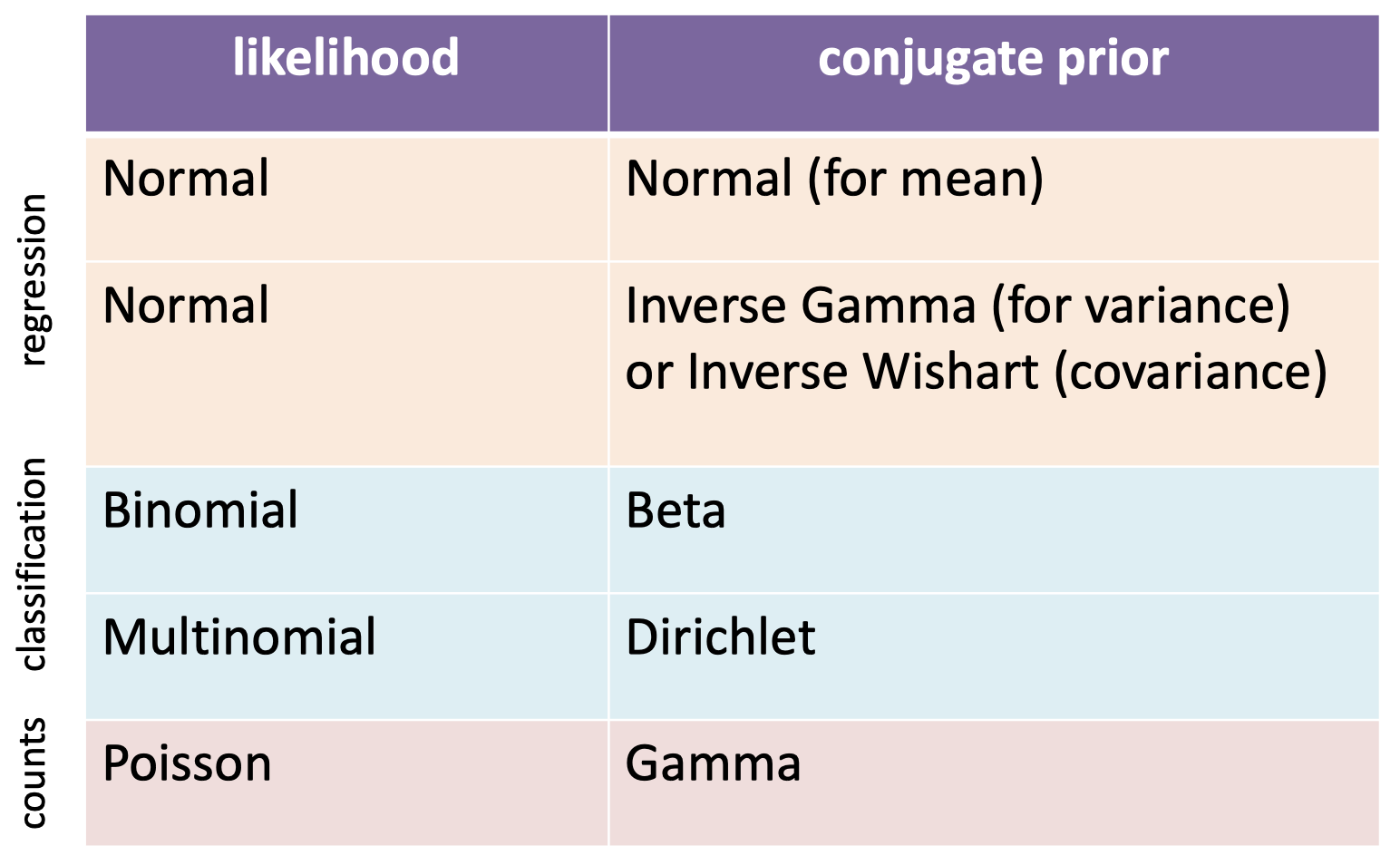## 2. 贝叶斯 Logistic 回归

### 2.1 再看 Logistic 回归

• 与回归问题相比，同样存在类似的参数不确定性问题
• 尽管内置了预测不确定性对输出建模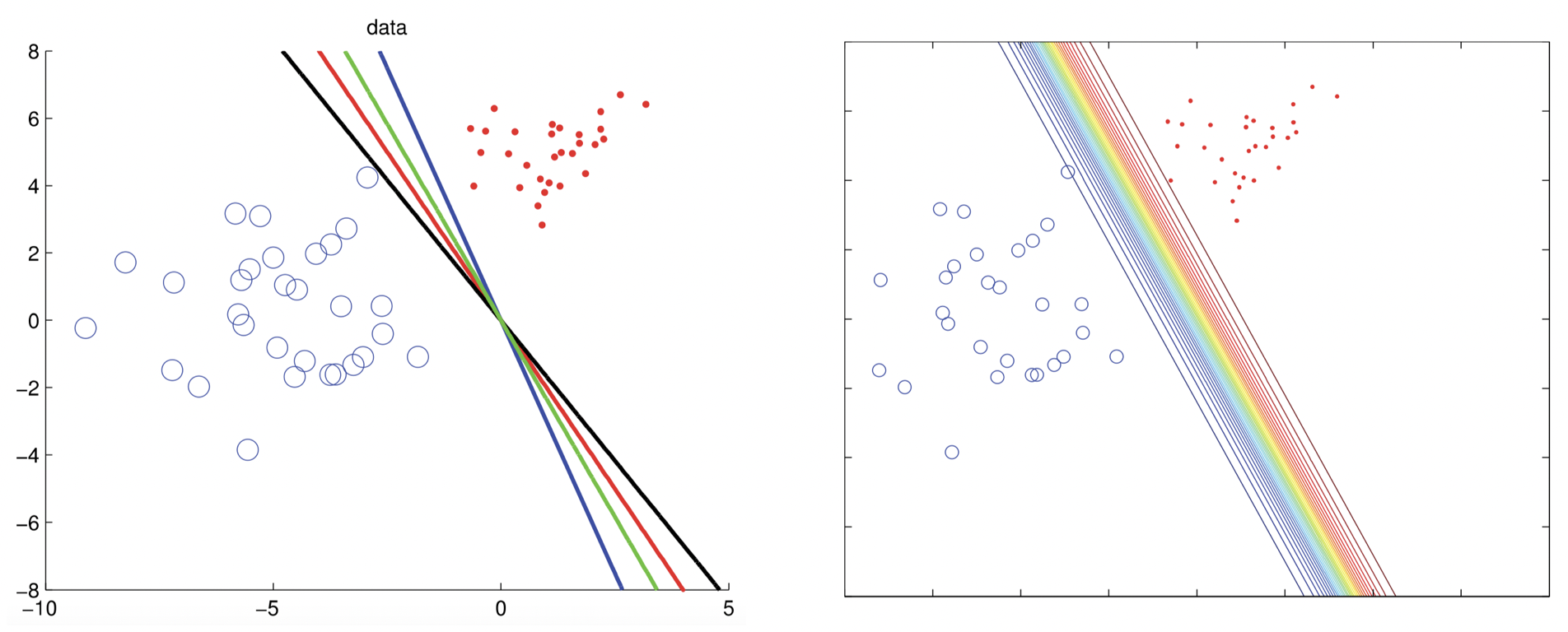Source: Machine Learning A Probabilistic Perspective, MLAPP (p.257-258) by Murphy

### 2.2 共轭不存在的情况

• 我们可以利用共轭先验吗？例如：
• 生成二分类模型（generative binary models）的 Beta-Binomial 共轭
• 多分类模型的 Dirichlet-Multinomial 共轭（类似的公式）
• 模型是 判别式的，Logistic 回归的参数由 sigmoid 函数定义（或者，对于多分类模型，参数由 softmax 函数定义；类似的问题和类似的解）

$p(y|q,\boldsymbol x)=q^y(1-q)^{1-y}$ $q=\sigma(\boldsymbol x'\boldsymbol w)$
• 需要 $\boldsymbol w$ 的先验，而不是 $q$
• 没有已知的共轭先验，所以使用高斯先验

### 2.3 近似

• 归一化常数没有已知的解

\begin{align} p(\boldsymbol w|\boldsymbol X,\boldsymbol y) &\propto p(\boldsymbol w)p(\boldsymbol y|\boldsymbol X,\boldsymbol w) \\ &= \text{Normal}(\boldsymbol 0,\sigma^2 \boldsymbol I)\prod_{i=1}^{n}\sigma(\boldsymbol x_i'\boldsymbol w)^{y_i}\left(1-\sigma(\boldsymbol x_i'\boldsymbol w)\right)^{1-y_i} \end{align}
• 通过近似求解
拉普拉斯近似：

• 假定众数的后验服从渐进正态
• 可以计算归一化常数、抽样等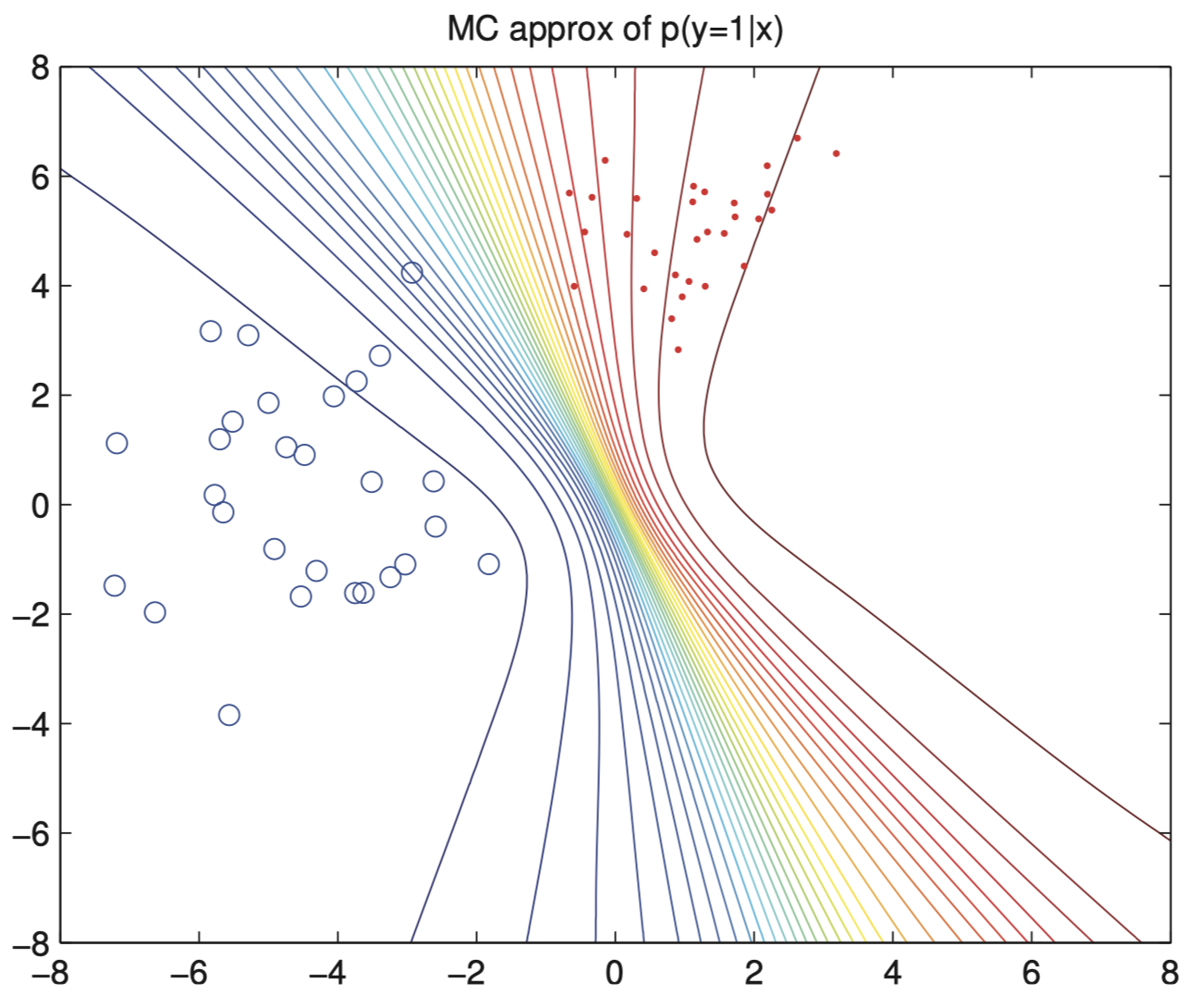Source: Machine Learning A Probabilistic Perspective, MLAPP (p.258) by Murphy

## 总结

• 离散设定下的贝叶斯思想
• Beta-Binomial 共轭
• 贝叶斯分类
• 非共轭的渐进必要性本作品采用知识共享署名-非商业性使用-相同方式共享 4.0 国际许可协议进行许可。 欢迎转载，并请注明来自：YEY 的博客 同时保持文章内容的完整和以上声明信息！